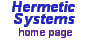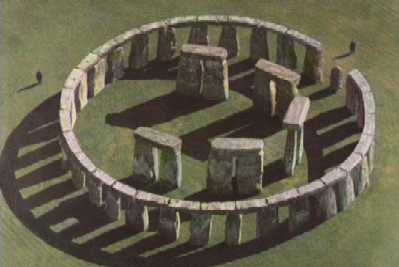The Dee-Cecil Calendar and itsDate Conversion Algorithms by Peter Meyer### Leap Year Rules

As stated in The Julian and Gregorian Calendars, in the Gregorian Calendar a year is a leap year if (and only if) either (i) its year number is divisible by 4 but not by 100 or (ii) it is divisible by 400. In other words, a year whose year number is divisible by 4 is a leap year unless it is divisible by 100 but not by 400 (in which case it is not a leap year). Thus the years 1600 and 2000 are leap years, but 1700, 1800, 1900 and 2100 are not. This rule is not all that difficult to understand, but is nevertheless beyond the comprehension of a significant proportion of the general population (and in any case, most people are ignorant of it, as can be established simply by asking a few).

The Gregorian Calendar has a 400-year cycle. In other words, the pattern of leap years (which are those years in which February has 29 days) repeats itself every 400 years. John Dee, an advisor to Queen Elizabeth I, proposed an alternative calendar (with the same structure of months and days as the Gregorian Calendar) based upon a 33-year cycle with a different rule for leap years, namely: A year is a leap year if (and only if) the year number, when divided by 33, yields a non-zero remainder which is a multiple of 4. For example, 2009 = 60*33 + 29; 29 is not an exact multiple of 4, so 2009 is not a leap year. 2012 = 60*33+32; 32 is an exact multiple of 4, so 2012 is a leap year. 2013 divided by 33 is exactly 61, so the remainder is zero, so 2013 is not a leap year. Thus in a 33-year cycle, the 4th, 8th, 12th, 16th, 20th, 24th, 28th and 32nd years are leap years (the others are not), so in a 33-year cycle there are exactly eight leap years (and 25*365 + 8*366 = 12,053 days).

The year 1 in this 33-year-cycle calendar is the first year of a 33-year cycle. This determines which years in the calendar are leap years. Thus some years (e.g., 2017) are leap years in this calendar but not in the Gregorian Calendar, and vice-versa. The pattern of combined leap years repeats after 33*400 = 13,200 years. Curiously, though, 13,200 Gregorian years (with 4,821,201 days) are one day longer than 13,200 Dee years (4,821,200 days). This is because the mean length of the year in the two calendars differs by 1/13,200.

In his Stonehenge Speaks: An Open Letter to Umberto Eco Simon Cassidy has described a method of ascertaining when a year is a leap year in this 33-year-cycle calendar without resorting to dividing by 33, as follows: Add the century number to the number of the year within the century (e.g., for 1996, 19 + 96 = 115). This may be greater than 99, in which case, repeat (e.g., 1 + 15 = 16). If the resulting number is greater than 33 then subtract 33 or 66 so that it is in the range 1 through 33. Then the year is a leap year if (and only if) this number is a multiple of 4 (so 1996 was a leap year).

### Accord with Vernal Equinox Year

As has been pointed out by Simon Cassidy and Duncan Steel, the average length of a seasonal year depends on the point in the Earth's orbit at which the year is taken to begin (vernal equinox, winter solstice, etc.). This is because, although the (quite small) ellipticity of the Earth's orbit is fairly constant, the axis of the ellipse is very slowly rotating. Thus the Earth is never in exactly the same position (with respect to the fixed stars) at two successive vernal equinoxes.

The mean vernal equinox year is not exactly the same as the mean winter solstice year, the mean autumnal equinox year or the mean summer solstice year (see How Britain got the Calendar Wrong). Since the Gregorian Calendar reform was meant to standardize the date of Easter, and the date of Easter depends on the date of the vernal equinox, the Gregorian calendar targets the vernal equinox year. The mean VE year is 365.24238 (or 365.2424 to four decimal places) days.

In the Gregorian Calendar there are, in every 400 calendar years, 97 leap years, so the average length of a year is (97*366 + 303*365)/400 = 365.2425 days exactly. In the 33-year calendar using the leap year rule given above, in every 33 years there are 8 leap years, so the average length of a year is (8*366 + 25*365)/33 = 365.24242... (repeating) days, which is closer to the mean VE year 365.24238 than is the mean Gregorian year 365.24250.

### Stonehenge as a 33-Year-Cycle CalendarReconstruction image © 1994 Simon Cassidy
According to the conceptual reconstruction (shown at right) of Stonehenge (as determined by Edgar Barclay in 1880) by Simon Cassidy (ca. 1983) and Mike Pitts (the archaeologist in charge of the 2005 full-size reconstruction on Salisbury Plain) there are 33 lintels (stones resting upon vertical stones), 5 capping the 5 trilithons in the center and 28 capping the stones in the surrounding sarsen circle.

In his Stonehenge Speaks article Simon Cassidy writes:

This reconstruction contains 73 stones total and 5 trilithons (central archways) possibly indicating the length of a normal year as 73 x 5 days (73 x 5 = 365). Note that there are 33 lintels (stones hung upon rooted stones) in two groups, a group of 28 lintels capping the circle and a group of 5 capping the trilithons. The solar calendrical significance of the lintels can indicate a 33-year leap-day cycle where a 366th. day is added seven times (every four years) in 28 years and then the eighth leap-day of the cycle is added after 5 more years.

Simon Cassidy has also explained how Stonehenge could have been used as an eclipse prediction device. See Re: Stonehenge et al (75-stone model).

### Correlation of Dates with Days

Dates in both calendars may be expressed as year-month-day, with years numbered according to the astronomical year numbering system (which allows a year 0 and negative years). The 33-year-cycle calendar is correlated with the empirical sequence of days by specifying a particular day, with a certain Julian day number JDN, as corresponding to the date 1-1-1. If JDN = 1,721,425 then the calendar is called the Dee Calendar. If JDN = 1,721,426 then the calendar is called the Dee-Cecil Calendar (after William Cecil, the treasurer of Queen Elizabeth I, who preferred removing ten days from the calendar rather than Dee's eleven days).

As stated above, the date 1-1-1 DC (that is, January 1st in the year 1 in the Dee-Cecil Calendar) denotes the day with Julian day number 1,721,426. This is the same day as 1-1-1 CE (that is, January 1st in the year 1 in the Common Era Calendar).

The Dee-Cecil Calendar may be considered to be a better candidate for calendar reform than the Dee Calendar for the reason that days back to March 1, 1980, and days forward to February 28, 2016, have the same dates in the Dee-Cecil Calendar as in the Gregorian. 2016 is a leap year in the Gregorian Calendar, but not in the Dee(-Cecil) Calendar. Consequently the day after February 28, 2016, is February 29 in the Gregorian Calendar but is March 1 in the Dee-Cecil Calendar.

### The Conversion Algorithms

To convert a date in a particular calendar to a date in another calendar it is sufficient to have two pairs of conversion algorithms. Each pair consists of (i) an algorithm for conversion of a calendar date (in one of the calendars) to its corresponding Julian day number and (ii) an algorithm for conversion of a Julian day number to the corresponding calendar date. This makes possible the conversion of dates in two calendars by passing through the corresponding Julian day numbers.

There already exist conversion algorithms for Gregorian (a.k.a. Common Era) calendar dates to and from Julian day numbers, so for conversion between dates in the Common Era Calendar and dates in the Dee-Cecil Calendar it is sufficient to provide conversion algorithms for dates in the Dee-Cecil Calendar to and from Julian day numbers. These are given below in pseudo-code, which provides a basis for their implementation in particular programming languages such as C and Visual Basic. They are preceded by two auxiliary functions.

The Mod operator is the usual modulus operator: A mod B = the remainder when A is divided by B, and \ is integer division: A \ B = the integer part of the result of dividing A by B. As used here, both operators need be defined only for integers A ≥ 0 and B ≥ 1.

The algorithms below make use of a quantity named JDN111. This is the Julian day number of 1-1-1. For the Dee Calendar this is defined as 1,721,425. For the Dee-Cecil Calendar it is defined as 1,721,426.

These algorithms were first published on this website on 2009-11-12.

#### When a year is a leap year

 ```Function name: IsDeeLeapYear Argument: Year Returns: True or False If Year >= 1 Then IsDeeLeapYear = ((((Year - 1) Mod 33) Mod 4) = 3) Else IsDeeLeapYear = (((-Year Mod 33) Mod 4) = 1) End If```

#### Number of days in a month

 ```Function name: NumDaysInDeeMonth Arguments: Year, Month Returns: Number of days If Month < 1 Or Month > 12 Then NumDaysInDeeMonth = 0 ElseIf Month = 2 Then If IsDeeLeapYear(Year) Then NumDaysInDeeMonth = 29 Else NumDaysInDeeMonth = 28 End If ElseIf Month = 4 Or Month = 6 Or Month = 9 Or Month = 11 Then NumDaysInDeeMonth = 30 Else NumDaysInDeeMonth = 31 End If```

#### Dee-Cecil date to Julian day number

 ```Function name: Dee2JDN Arguments: Year, Month, Day Returns: Julian day number or undefined If Not (Day >= 1 And Day <= NumDaysInDeeMonth(Month, Year)) Then Dee2JDN is undefined Exit Function End If 'Cycles consist of 33*365 + 8 = 12,053 days. 'Cycle 0 begins at 1-1-1. If Year >= 1 Then CycleNum = (Year - 1) \ 33 'cycle number Y = (Year - 1) Mod 33 'Y = year in cycle, 0-32 Else CycleNum = -(-Year \ 33) - 1 Y = 32 - ((-Year) Mod 33) End If X = CycleNum * 12053 + Y * 365 + (Y \ 4) 'Including addition of 1 for each leap year in cycle prior to year Y (Year). 'X is number of days since 1-1-1 of first day of year Y (Year). 'Add days in months. For M = 1 To Month - 1 X = X + NumDaysInDeeMonth(M, Year) Next Dee2JDN = X + Day + JDN111 - 1 'See above for value of JDN111.```

#### Julian day number to Dee-Cecil date

 ```Function name: JDN2Dee Argument: JDN (Julian day number) Returns: Year, Month, Day X = JDN - JDN111 'See above for value of JDN111. 'X is number of days since 1-1-1. If X >= 0 Then Year = 1 + (X \ 12053) * 33 D = X Mod 12053 'D is day number in 33-year cycle. Else Year = -(((-1 - X) \ 12053) * 33) - 32 D = 12052 - ((-X - 1) Mod 12053) End If For I = 1 To 33 If IsDeeLeapYear(I) Then NumDaysInYear = 366 Else NumDaysInYear = 365 End If If D < NumDaysInYear Then Exit For Else Year = Year + 1 D = D - NumDaysInYear End If Next 'Now D = day number in year. Month = 1 For I = 1 To 12 NumDaysInMonth = NumDaysInDeeMonth(I, Year) If D < NumDaysInMonth Then Exit For Else Month = Month + 1 D = D - NumDaysInMonth End If Next Day = D + 1```

### Date Conversion Software

The algorithms given above have been implemented in software for conversion of dates in the Julian, Gregorian, Dee and Dee-Cecil Calendars. See Julian-Gregorian-Dee Date Calculator.